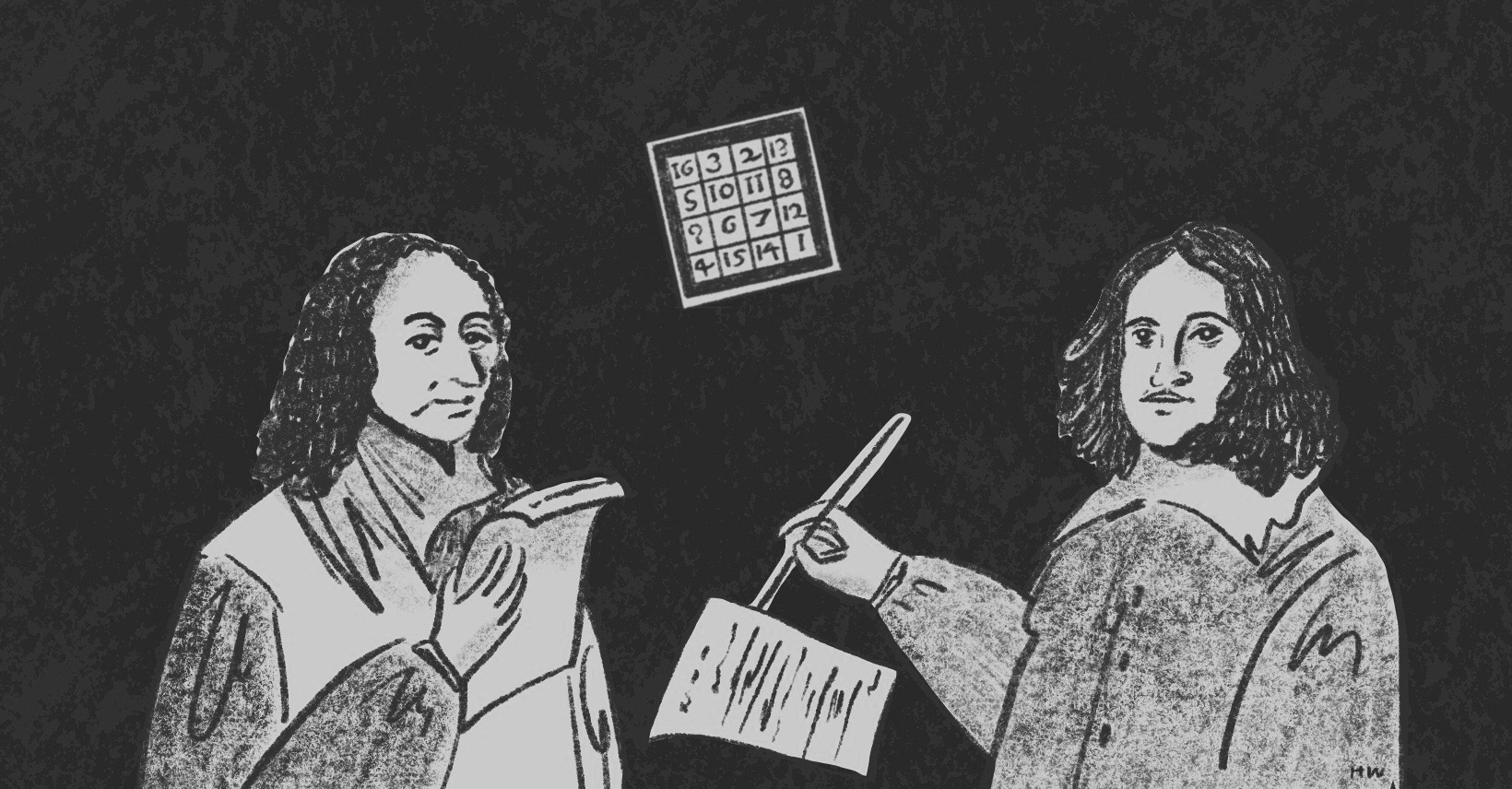×資料管理 統計分析 相關資源 巨人肩膀 語法索引 關於作者

## 簡介

• table() -交叉表
• summary() -輸出交叉表分析結果
• chisq.test() -卡方分析

$x^2=\displaystyle \sum_{_i=1}^{r}\displaystyle \sum_{_j=1}^{c}\frac{(O_{i,j}-E_{i,j})^2}{E_{i,j}}$

$$O$$= Observer觀察值。

$$E$$= Expected value期望值。

$$\displaystyle \sum_{i=1}^{r}$$, $$\displaystyle \sum_{i=1}^{c}$$= 加總列(r)與欄(c)觀察值與期望值的差異。

## 卡方分析

Number of Crash Involvements in Florida, 1987
Model Year Rear Impacts Others (Control Group)
1985 (without CHMSL) 6,773 22,959
1986 (CHMSL-equipped) 7161 25,989

 > thirdlight_tab<-matrix(c(6773, 22959, 7161, 25989), ncol=2, byrow=TRUE) #建立矩陣 > colnames(thirdlight_tab)<-c("Rear Impacts", "Others") #命名矩陣的欄 > rownames(thirdlight_tab)<-c("1985 (without)", "1986 (equipped)") #命名矩陣的列 > thirdlight_tab<-as.table(thirdlight_tab) #將矩陣轉換為交叉表 > thirdlight_tab #輸出交叉表 Rear Impacts Others 1985 (without) 6773 22959 1986 (equipped) 7161 25989 > summary(thirdlight_tab) #輸出卡方分析結果 Number of cases in table: 62882 Number of factors: 2 Test for independence of all factors: Chisq = 12.618, df = 1, p-value = 0.0003821 

 > chisq.test(thirdlight_tab) Pearson's Chi-squared test with Yates' continuity correction data: thirdlight_tab X-squared = 12.549, df = 1, p-value = 0.0003963 

## 期望值

 > addmargins(thirdlight_tab) #邊際加總 Rear Impacts Others Sum 1985 (without) 6773 22959 29732 1986 (equipped) 7161 25989 33150 Sum 13934 48948 62882 

Model Year Rear Impacts Others (Control Group) Sum
1985 (without) 6773 (期望值6588) 22959 (期望值23144) 29732
1986 (equipped) 7161 (期望值7376) 25989 (期望值25804) 33150
Sum 13934 48948 62882

 > chisq<-chisq.test(thirdlight_tab) #將卡方分析結果指定給chisq > round(chisq\$expected) #計算期望值並四捨五入 Rear Impacts Others 1985 (without) 6588 23144 1986 (equipped) 7346 25804 

 > (((6773-6588)^2)/6588)+(((22959-23144)^2)/23144)+(((7161-7346)^2)/7346)+(((25989-25804)^2)/25804) 

$1-\frac{\tfrac{7161}{6773}}{\tfrac{25989}{22959}}=0.0659$